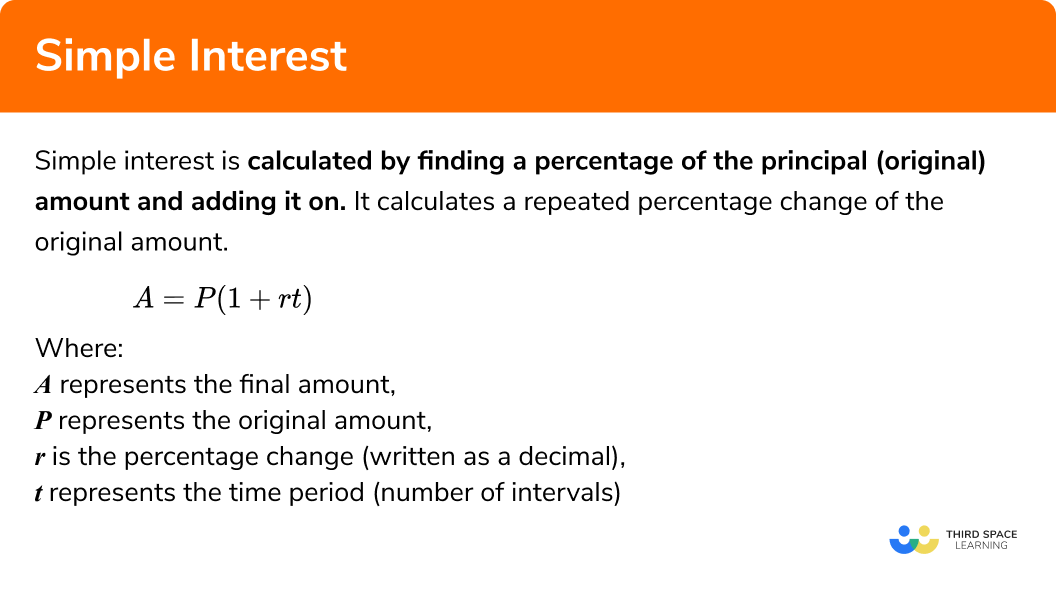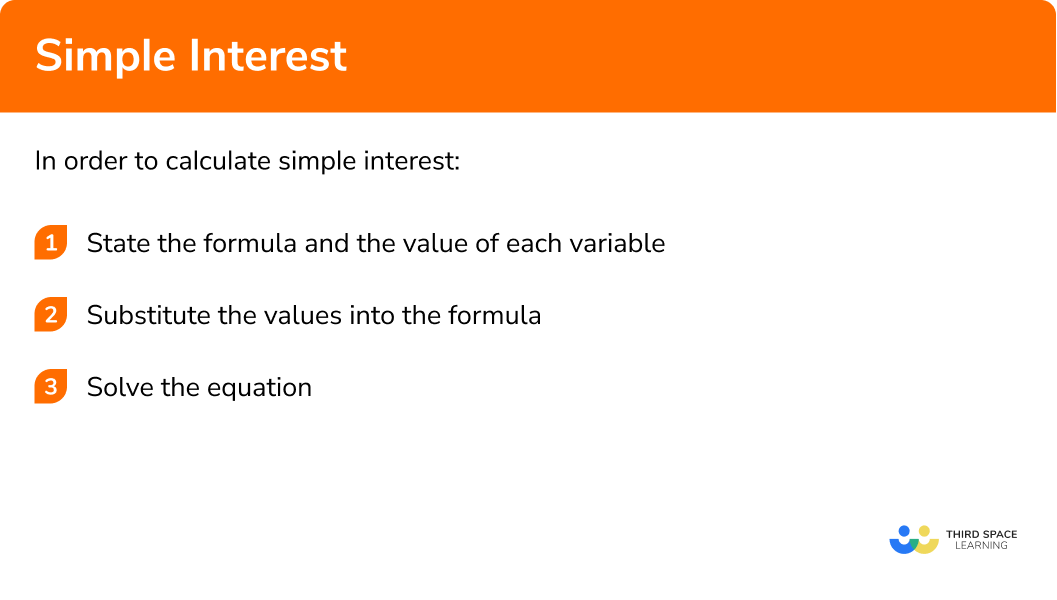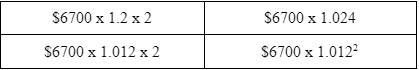# Simple Interest

Here we will learn about simple interest including how to calculate simple interest for increasing and decreasing values, and how to set up, solve and interpret growth and decay problems.

There are also simple interest worksheets based on Edexcel, AQA and OCR exam questions, along with further guidance on where to go next if you’re still stuck.

## What is simple interest?

Simple interest is calculated by finding a percentage of the principal (original) amount and multiplying by the time period of the investment. The final value of the investment can then be found by adding the simple interest to the principal amount.

Simple Interest Formula

Simple interest can be calculated using the following formula:

I=Prt

And we can calculate the value of the investment, A, after the time period with the formula:

\begin{aligned} A& =P+Prt \\\\ & =P\left( 1+rt \right) \end{aligned}

Where:

• I represents the simple interest
• A represents the final amount.
• P represents the original amount.
• r is the percentage rate (written as a decimal).
• t represents the time period (number of intervals).

E.g

Lets calculate the interest earned on £3000 with a simple interest rate of 5\% over 2 years.

Using the formula I=Prt, remembering that 5\% = 0.05

\begin{aligned} I&=3000\times 0.05\times 2 \\\\ & =\text{£}300 \end{aligned}

To find the final value of the investment we can now add the interest to the principal amount.

\begin{aligned} A&=3000+300 \\\\ & =\text{£}3300 \end{aligned}

We could have calculated this directly using the formula A=P\left( 1+rt \right)

\begin{aligned} A& =3000\left( 1+0.05\times 2 \right) \\\\ & =\text{£}3300 \end{aligned}

### What is simple interest?## How to calculate simple interest

In order to calculate simple interest:

1. State the formula needed and the value of each variable.
2. Substitute the values into the formula.
3. Solve the equation.

### Explain how to calculate simple interest in 3 steps.Simple interest is part of our series of lessons to support revision on simple interest and compound interest. You may find it helpful to start with the main simple interest and compound interest lesson for a summary of what to expect, or use the step by step guides below for further detail on individual topics. Other lessons in this series include:

## Simple interest examples

### Example 1: Finding the simple interest

£2100 is invested for 3 years at an annual interest rate of 2\% per year simple interest. Find the interest earned on the investment in that time?

1. State the formula needed and the value of each variable

We required the interest so we will use the formula I=Prt with:

• P=2100
• r=0.02
• t=3

2Substitute the values into the formula.

Substituting these values into the simple interest formula I=Prt, we get:

I=2100\times 0.02\times 3

3Solve the equation.

I=£126

£126 was earned on the investment.

### Example 2: Simple interest –  finding the final amount after an increase

£1500 is invested for 4 years at an annual interest rate of 5\% per year simple interest. What is the value of the investment after this time?

We required final the value of the investment so we will use the formula A=P(1+rt) with:

• P=1500
• r=0.05
• t=4

Substituting these values into the simple interest formula A=P(1+rt), we get:

A=1500(1+0.05\times{4})

\begin{aligned} &A=1500(1+0.20)\\\\ &A=1500(1.20)\\\\ &A=1500\times{1.2}\\\\ &A=\pounds1800. \end{aligned}

### Example 3: Simple interest – finding the final amount after a decrease

A car is bought for £10,000 and loses 9\% of its value per annum, simple interest. What is the value of the car after 8 years?

Here we use the formula A=P(1+rt) with:

• P=10000
• r=-0.09
• t=8

Substituting these values into the simple interest formula A=P(1+rt), we get:

A=10000(1-0.09\times{8})

\begin{aligned} &A=10000(1-0.72)\\\\ &A=10000(0.28)\\\\ &A=10000\times{0.28}\\\\ &A=\pounds2800. \end{aligned}

### Example 4: Simple interest – different time scale

£7600 is borrowed for 2 years on a credit card. The cost of borrowing is a 1\% interest payment per month simple interest for the life of the loan. What is the total cost to pay off after this time?

Here we use the formula A=P(1+rt) with:

• P=7600
• r=0.01
• t= 2 \times 12 = 24 (remember there are 12 months in 1 year)

Substituting these values into the simple interest formula A=P(1+rt), we get:

A=7600(1+0.01\times{24})

\begin{aligned} &A=7600(1+0.24)\\\\ &A=7600(1.24)\\\\ &A=7600\times{1.24}\\\\ &A=\pounds{9424}. \end{aligned}

### Example 5: Simple interest – different percentages

A house is currently valued at £175,000. For the first 3 years, the value of the house increases by the rate of simple interest of 0.2\% per annum. For the following 4 years, the value of the house decreases in value by a simple interest rate of 0.18\% per annum. Calculate the value of the house after these 7 years.

Here we use the formula

A=P\left(1+r_{1} t_{1}+r_{2} t_{2}\right)

• P=175,000
• r_{1}=0.002
• t_{1}=3
• r_{2}=-0.0018
• t_{2}=4

Substituting these values into the simple interest formula

A=P\left(1+r_{1} t_{1}+r_{2} t_{2}\right),

we get:

A=175,000(1+0.002\times{3}-0.0018\times{4})

\begin{aligned} &A=175,000(1+0.006-0.0072)\\\\ &A=175,000(0.9988)\\\\ &A=175,000\times{0.9988}\\\\ &A=\pounds{174,790}. \end{aligned}

### Common misconceptions

• Applying the incorrect formula to the question

This is a very common mistake where the simple interest on an amount is calculated instead of using the compound interest formula.

• Incorrect percentage change due to different time scales

In example 4 “ £7600 is invested for 2 years at 1\% per month simple interest. What is the value of the investment after this time?”, the value of t is written as 2 and not 24.

Here we use the formula A=P(1+rt) with:

• P=7600
• r=0.01
• t= 2

Substituting these values into the simple interest formula A=P(1+rt), we get:

A=7600(1+0.01\times{2})

\begin{aligned} &A=7600(1+0.02)\\\\ &A=7600(1.02)\\\\ &A=7600\times{1.02}\\\\ &A=\pounds{7752}. \end{aligned}

• Using the incorrect value for the percentage change

Using the percentage as the value for r (therefore not dividing the percentage by 100 ). For example when using simple interest to increase £100 by 2\% for 5 years, this calculation is made:

\begin{aligned} &A=100(1+2\times{5})\\\\ &A=100\times{11}\\\\ &A=\pounds{1100!} \end{aligned}

• Is the value increasing or decreasing?

If a value is depreciating (going down), the value of r is negative whereas it is incorrectly used as a positive and so the answer will be larger than the original amount.

### Simple Interest practice questions

1. A technology store has a back to school offer: save 20\% on all full price laptops. Paula buys a laptop that was £689 full price. After 3 years, the value of the purchased laptop has decreased by 4\% per year, simple interest. What is the value of the laptop after these 3 years?

£551.20£485.06£529.15£507.10The original price was £689 . The sale price was 80 % of this. 80 % of £689 is £551.20 . The value of the laptop has decreased by 4 % of £551.20 per year for three years. We can work out 4 % of 551.20 and multiply by 3 ; this is £66.14 . Subtracting this from the purchase price gives the new value.

2. A saxophone that costs £999 is bought using a finance deal. The total price is divided by the number of months with 7% of the original amount added as an extra charge. The finance deal is spread over 2 years. How much money has been spent on the saxophone after 6 months?

£1068.93£801.70£44.54£267.23The amount to be paid back is given by 1.07\times999=1068.93
Six months is a quarter of the finance period, so a quarter of the full amount will have been spent on the saxophone, therefore \frac{1}{4}\times1068.93=267.23

3. Two shops have a sale. Shop A advertises a 10 % reduction on all items. Shop B has reduced all items by 8 % with a further 2 % reduction on the sale price of t-shirts. Josie can buy the same t-shirt from each shop that originally costs £16 . Which shop is selling the item cheapest?

Shop AShop BShop A: After a 10 % reduction, the t-shirt will cost £14.40
Shop B: The 8 % reduction means the t-shirt will be £14.72 , with the additional 2 % reduction making the price £14.43

4. £7342 is invested in a savings account with a 0.4 % simple interest rate per month. What is the value of the investment after 4 years?

£7459.47£7694.42£8751.66£8046.83The initial amount is 7342 , the simple interest rate is 0.4 % and the number of months is 48 , which results in 7342(1+0.004\times48)

5. A house is valued at £365,500 . The value of the house increases by an average of 0.25 % per year, simple interest. How much is the house worth after 12 years?

£366,413£376,465£497,080£387,430The initial amount is 365500 , the simple interest rate is 0.25 % and the number of years is 12 , which results in 365500(1+0.0025\times12)

### Simple Interest GCSE Exam Questions:

1.(a) £850 is invested for 6 years at 2\% simple interest per year. Work out the total interest earned?

(b) The interest rate changes to 0.15\% simple interest per month. How does this affect the final value of the investment over the same time period of 6 years?

(6 marks)

(a)

850 \times 0.02 \times 6

(1)

£102

(1)

(b)

0.15 \times 12 \times 6 = 10.8\%

(1)

850 \times (0.108)

(1)

£91.80

(1)

Decreased by £10.20 over 6 years

(1)

2. (a) Freya invests \$6700 for 2 years. The simple interest rate is 1.2\% per year. Which calculation below works out the total value after 2 years? Circle your answer.(b) Euan invests \$6400 for 2 years. The simple interest rate is

1.4\% for the first year

1.1\% for the second year.

Whose investment is worth more after 2 years and by how much?

(4 marks)

(a) \$6700 \times 1.024 (1) (b) 1.4+1.1=2.5\% (1) \$6700 \times (0.001)

(1)

\\$6.70

(1)

3. (a) Lauren places £300 into a new bank account with a simple interest rate of 3\%. After the second year, she withdraws £30. How much money would she have after 5 years?

(b) The interest rate after 5 years halves. How many years will it now take Lauren to save £400?

(5 marks)

(a)

300 \times (1+0.03 \times 5)-30

(1)

£315

(1)

(b)

400-315=£85

(1)

85 \div 4.725

(1)

18 years

(1)

## Learning checklist

You have now learned how to:

• Solve problems involving percentage change, including: percentage increase, decrease and original value problems and simple interest in financial mathematics
• Set up, solve and interpret the answers in growth and decay problems, including compound interest {and work with general iterative processes}

## Still stuck?

Prepare your KS4 students for maths GCSEs success with Third Space Learning. Weekly online one to one GCSE maths revision lessons delivered by expert maths tutors.

Find out more about our GCSE maths tuition programme.